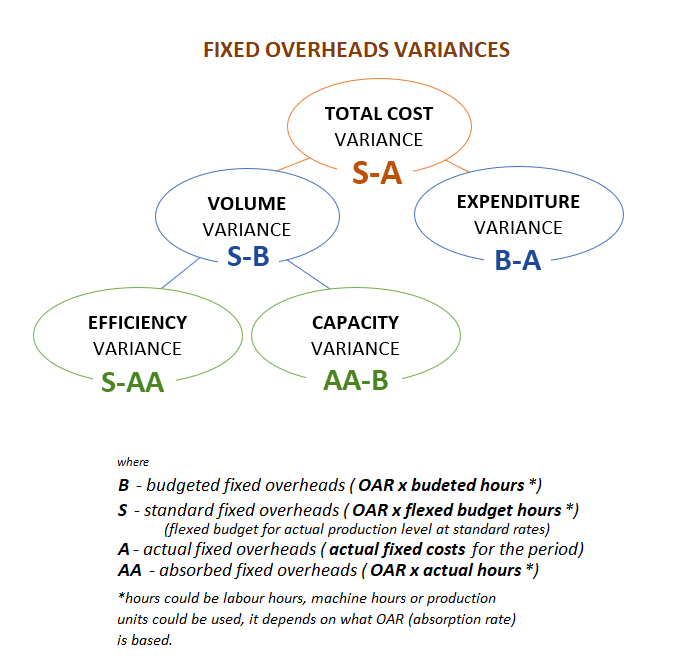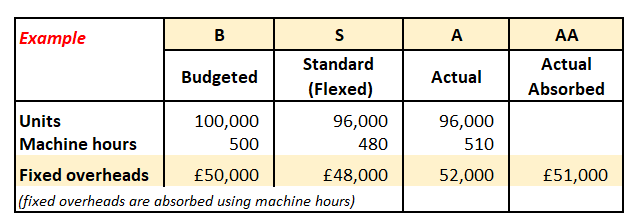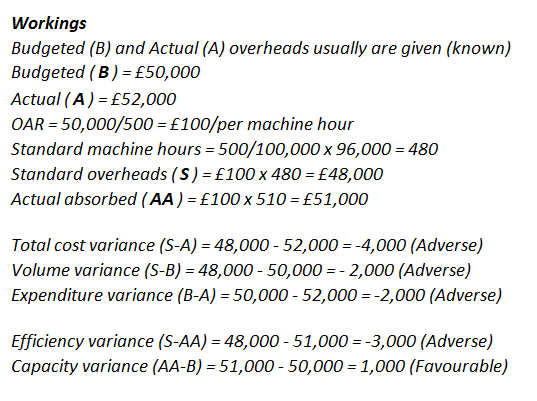Hi everyone,

After 2 days of trying to remember all the formula used to calculate the variances. I remembered what our professor was saying in the university: "Mathematics do not have to remember they have to get it using the logic".

And I found the logic in fixed overhead calculations. Just remember easy formulas S-A = (S-B) + (B-A) and S-B = (S-AA) + (AA-B), where
B and A are usually known, Standard overheads = OAR x standard hours (calculated using flexing budget rules), AA = actual hours x OAR

•••• East YorkshireRegistered, Tutor Posts: 53
That's one way . . .

I prefer - Total OH Variance is the difference between what it did cost and what it should have cost.

This total variance breaks down into expenditure variance (which ignores the 'should have' bit) and volume variance.

Volume then breaks down again into efficiency and capacity.

Capacity Variance is the one that usually causes problems as it is not so instinctive. If we actually use fewer hours than the original base budget, this is Adverse because we ae not fully using those assets we have invested in.
• Hi, mcchoc. Thank you for your comment! How do you remember how to calculate all these variances?
• Registered, Tutor Posts: 165
As mcchoc says, look at the difference between what should happen and what actually happened.

In your example, each unit should take 0.005 hours (500/100,000) and you recover £100 overhead per hour (£50,000/500)

So, 96,000 units should take 480 hours (96,000 x 0.005)
They actually took 510 hours, 30 hours too long
This is an efficiency problem, which reduces your overhead recovery by £3,000 (30 hrs x £100/hr)
• East YorkshireRegistered, Tutor Posts: 53
Foolproof method for Materials and Labour variances

Create three columns BudgetActualStandard

Under each title, tear the data to shreds, ie kg/unit, £/kg, total Kg, total material cost; hours/unit, £/labour hour, total labour hours, total labour cost.

Remember - the Standard Budget is just the original budget flexed by the new Volume, so we flex the quantities of materials and labour hours, but NOT the unit price.